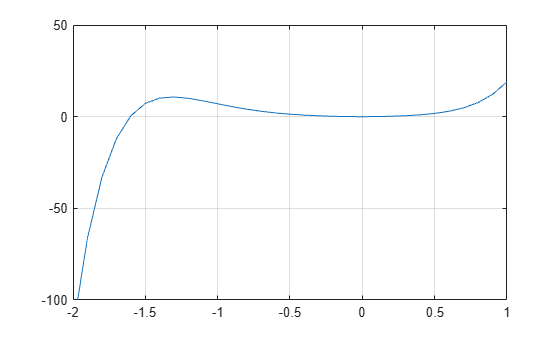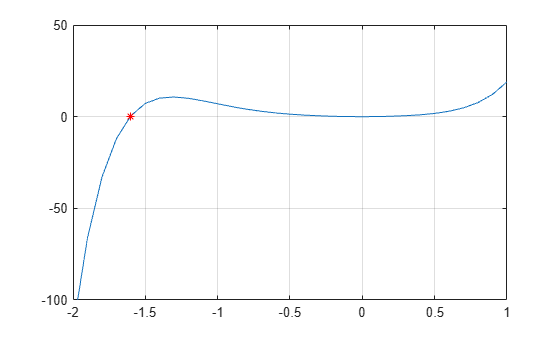Documentation

## Roots of Polynomials

This example shows several different methods to calculate the roots of a polynomial.

### Numeric Roots

The `roots` function calculates the roots of a single-variable polynomial represented by a vector of coefficients.

For example, create a vector to represent the polynomial ${x}^{2}-x-6$, then calculate the roots.

```p = [1 -1 -6]; r = roots(p) ```
```r = 3 -2```

By convention, MATLAB® returns the roots in a column vector.

The `poly` function converts the roots back to polynomial coefficients. When operating on vectors, `poly` and `roots` are inverse functions, such that `poly(roots(p))` returns `p` (up to roundoff error, ordering, and scaling).

```p2 = poly(r) ```
```p2 = 1 -1 -6```

When operating on a matrix, the `poly` function computes the characteristic polynomial of the matrix. The roots of the characteristic polynomial are the eigenvalues of the matrix. Therefore, `roots(poly(A))` and `eig(A)` return the same answer (up to roundoff error, ordering, and scaling).

### Roots Using Substitution

You can solve polynomial equations involving trigonometric functions by simplifying the equation using a substitution. The resulting polynomial of one variable no longer contains any trigonometric functions.

For example, find the values of $\theta$ that solve the equation

`$3{\mathrm{cos}}^{2}\left(\theta \right)-\mathrm{sin}\left(\theta \right)+3=0.$`

Use the fact that ${\mathrm{cos}}^{2}\left(\theta \right)=1-{\mathrm{sin}}^{2}\left(\theta \right)$ to express the equation entirely in terms of sine functions:

`$-3{\mathrm{sin}}^{2}\left(\theta \right)-\mathrm{sin}\left(\theta \right)+6=0.$`

Use the substitution $x=\mathrm{sin}\left(\theta \right)$ to express the equation as a simple polynomial equation:

`$-3{x}^{2}-x+6=0.$`

Create a vector to represent the polynomial.

`p = [-3 -1 6];`

Find the roots of the polynomial.

`r = roots(p)`
```r = 2×1 -1.5907 1.2573 ```

To undo the substitution, use $\theta ={\mathrm{sin}}^{-1}\left(x\right)$. The `asin` function calculates the inverse sine.

`theta = asin(r)`
```theta = 2×1 complex -1.5708 + 1.0395i 1.5708 - 0.7028i ```

Verify that the elements in `theta` are the values of $\theta$ that solve the original equation (within roundoff error).

```f = @(Z) 3*cos(Z).^2 - sin(Z) + 3; f(theta)```
```ans = 2×1 complex 10-14 × -0.0888 + 0.0647i 0.2665 + 0.0399i ```

### Roots in a Specific Interval

Use the `fzero` function to find the roots of a polynomial in a specific interval. Among other uses, this method is suitable if you plot the polynomial and want to know the value of a particular root.

For example, create a function handle to represent the polynomial $3{x}^{7}+4{x}^{6}+2{x}^{5}+4{x}^{4}+{x}^{3}+5{x}^{2}$.

`p = @(x) 3*x.^7 + 4*x.^6 + 2*x.^5 + 4*x.^4 + x.^3 + 5*x.^2;`

Plot the function over the interval $\left[-2,1\right]$.

```x = -2:0.1:1; plot(x,p(x)) ylim([-100 50]) grid on hold on```From the plot, the polynomial has a trivial root at `0` and another near `-1.5`. Use `fzero` to calculate and plot the root that is near `-1.5`.

`Z = fzero(p, -1.5)`
```Z = -1.6056 ```
`plot(Z,p(Z),'r*')`### Symbolic Roots

If you have Symbolic Math Toolbox™, then there are additional options for evaluating polynomials symbolically. One way is to use the `solve` function.

```syms x s = solve(x^2-x-6) ```
```s = -2 3```

Another way is to use the `factor` function to factor the polynomial terms.

```F = factor(x^2-x-6) ```
```F = [ x + 2, x - 3]```